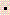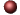Chemical Reactions and Reaction StoichiometryExamples ofMultiple Choice Questions

1.
Balance the following equation with the smallest whole number coefficients. Choose the answer that is the sum of the coefficients in the balanced equation. Do not forget coefficients of "one."
PtCl4 + XeF2PtF6 + ClF + Xe

(a) 16
(b) 22
(c) 24
(d) 26
(e) 32

2.
Balance the following equation with the smallest whole number coefficients. Choose the answer that is the sum of the coefficients in the balanced equation. Do not forget coefficients of "one."
Cr2(SO4)3 + RbOHCr(OH)3 + Rb2SO4

(a) 10
(b) 12
(c) 13
(d) 14
(e) 15

3.
Balance the following equation using minimum integral coefficients:
NH3 + O2NO2 + H2O

The stoichiometric coefficient for oxygen gas O2 is:
(a) 1
(b) 4
(c) 3
(d) 7
(e) 5

4.
When iron pyrite (FeS2) is heated in air, the process known as "roasting" forms sulfur dioxide and iron(III) oxide. When the equation for this process is completed and balanced, using the smallest whole number coefficients, what is the coefficient for "O2"?
___ FeS2 + ___ O2___ SO2 + ___ Fe2O3

(a) 2
(b) 4
(c) 7
(d) 8
(e) 11

5.
How many moles of KBrO3 are required to prepare 0.0700 moles of Br2 according to the reaction:
KBrO3 + 5KBr + 6HNO36KNO3 + 3Br2 + 3H2O

(a) 0.210
(b) 0.0732
(c) 0.0704
(d) 0.220
(e) 0.0233

6.
Which of the following statements is FALSE for the chemical equation given below in which nitrogen gas reacts with hydrogen gas to form ammonia gas assuming the reaction goes to completion?
N2 + 3H22NH3

(a) The reaction of one mole of H2 will produce 2/3 moles of NH3.
(b) One mole of N2 will produce two moles of NH3.
(c) One molecule of nitrogen requires three molecules of hydrogen for complete reaction.
(d) The reaction of 14 g of nitrogen produces 17 g of ammonia.
(e) The reaction of three moles of hydrogen gas will produce 17 g of ammonia.

7.
Calcium carbide, CaC2, is an important preliminary chemical for industries producing synthetic fabrics and plastics. CaC2 may be produced by heating calcium oxide with coke:
CaO + 3CCaC2 + CO

What is the amount of CaC2 which can be produced from the reaction of excess calcium oxide and 10.2 g of carbon? (Assume 100% efficiency of reaction for purposes of this problem.)

(a) 18.1 g
(b) 28.4 g
(c) 20.8 g
(d) 19.8 g
(e) 27.2 g

8.
Calculate the mass of hydrogen formed when 25 grams of aluminum reacts with excess hydrochloric acid.
2Al + 6HClAl2Cl6 + 3H2

(a) 0.41 g
(b) 1.2 g
(c) 1.8 g
(d) 2.8 g
(e) 0.92 g

9.
When 12 g of methanol (CH3OH) was treated with excess oxidizing agent (MnO4-), 14 g of formic acid (HCOOH) was obtained. Using the following chemical equation, calculate the percent yield. (The reaction is much more complex than this; please ignore the fact that the charges do not balance.)
3CH3OH + 4MnO4-3HCOOH + 4MnO2

(a) 100%
(b) 92%
(c) 82%
(d) 70%
(e) 55%

10.
A commercially valuable paint and adhesive stripper, dimethyl sulfoxide (DMSO), (CH3)2SO, can be prepared by the reaction of oxygen with dimethyl sulfide, (CH3)2S, using a ratio of one mole oxygen to two moles of the sulfide:
O2 + 2(CH3)2S2(CH3)2SO

If this process is 83% efficient, how many grams of DMSO could be produced from 65 g of dimethyl sulfide and excess O2?
(a) 68 g
(b) 75 g
(c) 83 g
(d) 51 g
(e) 47 g

11.
The formation of ethyl alcohol (C2H5OH) by the fermentation of glucose (C6H12O6) may be represented by:
C6H12O62C2H5OH + 2CO2

If a particular glucose fermentation process is 87.0% efficient, how many grams of glucose would be required for the production of 51.0 g of ethyl alcohol (C2H5OH)?

(a) 68.3 g
(b) 75.1 g
(c) 115 g
(d) 229 g
(e) 167 g

12.
The limiting reagent in a chemical reaction is one that:
(a) has the largest molar mass (formula weight).
(b) has the smallest molar mass (formula weight).
(c) has the smallest coefficient.
(d) is consumed completely.
(e) is in excess.

13.
If 5.0 g of each reactant were used for the the following process, the limiting reactant would be:
2KMnO4 +5Hg2Cl2 + 16HCl10HgCl2 + 2MnCl2 + 2KCl + 8H2O

(a) KMnO4
(b) HCl
(c) H2O
(d) Hg2Cl2
(e) HgCl2

14.
What mass of ZnCl2 can be prepared from the reaction of 3.27 grams of zinc with 3.30 grams of HCl?
Zn +2HClZnCl2 + H2

(a) 6.89 g
(b) 6.82 g
(c) 6.46 g
(d) 6.17 g
(e) 6.02 g

15.
How many grams of NH3 can be prepared from 77.3 grams of N2 and 14.2 grams of H2? (Hint: Write and balance the equation first.)

(a) 93.9 g
(b) 79.7 g
(c) 47.0 g
(d) 120.0 g
(e) 13.3 g

16.
Silicon carbide, an abrasive, is made by the reaction of silicon dioxide with graphite.
SiO2 +3CSiC + 2CO

If 100 g of SiO2 and 100 g of C are reacted as far as possible, which one of the following statements will be correct?

(a) 111 g of SiO2 will be left over.
(b) 44 g of SiO2 will be left over.
(c) 82 g of C will be left over.
(d) 40 g of C will be left over.
(e) Both reactants will be consumed completely, with none of either left over.

17.
Calculate the mass of 6.00% NiSO4 solution that contains 40.0 g of NiSO4?
(a) 667 g
(b) 540 g
(c) 743 g
(d) 329 g
(e) none of these

18.
How many grams of water are contained in 75.0 grams of a 6.10% aqueous solution of K3PO4?
(a) 75.0 g
(b) 73.2 g
(c) 70.4 g
(d) 68.1 g
(e) 62.8 g

19.
The mass (in grams) of FeSO47H2O required for preparation of 125 mL of 0.90 M solution is:
(a) 16 g
(b) 25 g
(c) 13 g
(d) 31 g
(e) 43 g

20.
What is the molarity of phosphoric acid in a solution labeled 20.0% phosphoric acid (H3PO4) by weight with a density = 1.12 g/mL?

(a) 0.98 M
(b) 2.3 M
(c) 2.7 M
(d) 3.0 M
(e) 3.6 M

21.
How many mL of 17 M NH3 must be diluted to 500.0 mL to make a 0.75 M solution?
(a) 13 mL
(b) 22 mL
(c) 39 mL
(d) 73 mL
(e) none of these

22.
How many grams of Ag2CO3 are required to react with 28.5 mL of 1.00 M NaOH solution?
Ag2CO3 +2NaOHAg2O + Na2CO3 + H2O
(a) 7.87 g
(b) 3.93 g
(c) 15.7 g
(d) 10.8 g
(e) 8.16 g

23.
How many milliliters of 0.200 M NH4OH are needed to react with 12.0 mL of 0.550 M FeCl3?
FeCl3 + 3NH4OHFe(OH)3 + 3NH4Cl

(a) 99.0 mL
(b) 33.0 mL
(c) 8.25 mL
(d) 68.8 mL
(e) 132 mL

24.
When 250. mL of a 0.15 M solution of ammonium sulfide (NH4)2S is poured into 120. mL of a 0.053 M solution of cadmium sulfate CdSO4, how many grams of a yellow precipitate of cadmium sulfide CdS are formed? The other product is (NH4)2SO4. (Hint: Write out and balance the equation. Is this a limiting reagent problem? )

(a) 5.4 g
(b) 0.92 g
(c) 2.6 g
(d) 1.9 g
(e) 530 g

1. (a) 2. (b) 3. (d) 4. (e) 5. (e) 6. (e) 7. (a) 8. (d) 9. (c) 10. (a) 11. (c) 12. (d) 13. (d) 14. (d) 15. (b) 16. (d) 17. (a) 18. (c) 19. (d) 20. (b) 21. (b) 22. (b) 23. (a) 24. (b)Click here to return to the top.Choose your next chapter:
Fundamentals of Chemistry | Chemical Formulas & Composition Stoichiometry | Chemical Equations & Rxn Stoichiometry | Types of Chemical Reactions |
| Atomic Structure | Chemical Periodicity | Chemical Bonding | Molecular Structure/Covalent Bonding Theories| Molecular Orbital Theory |
| Acids/Bases/Salts - Theory & Rxns | Acids/Bases/Salts - Calculations (including balancing redox rxns) | Gases | Solids & Liquids | Solutions |
| Thermodynamics | Kinetics | Equilibrium | Aqueous Equilibrium - Acids/Bases/Salts | Aqueous Equilibrium - Buffers & Titrations |
| Aqueous Equilibrium - Slightly Soluble Salts | Electrochemistry | Metallurgy | Metal Properties & Rxns | Nonmetals & Metalloids |
| Coordination Compounds | Nuclear Chemistry | Organic Chem - Formulas/Names/Properties | Organic Chem - Shapes/Rxns/Biopolymers |To report any corrections, please e-mail Dr. Wendy Keeney-Kennicutt.